# SAS Tutorials: Subsetting and Splitting Datasets

This SAS software tutorial shows how to subset or split a dataset from within a data step.

## Subsetting vs. Splitting

When preparing data for analysis, you may need to "filter out" cases (rows) from your dataset, or you may need to divide a dataset into separate pieces. In this tutorial, we use the following terms to refer to these two tasks:

A subset is selection of cases taken from a dataset that match certain criteria. You can also think of this as "filtering" a dataset so that only some cases are included. When subsetting a dataset, you will only have a single new dataset as a result.

A split acts as a partition of a dataset: it separates the cases in a dataset into two or more new datasets. When splitting a dataset, you will have two or more datasets as a result.

Both subsetting and splitting are performed within a data step, and both make use of conditional logic. Both processes create new datasets by pulling information out of an existing dataset based on certain criteria. The difference between the two processes is in how the cases are selected.

Note: A related task is to select a subset of variables (columns) from a dataset. For instructions on how to drop or keep variables from a dataset, see our Data Step tutorial.

## Subsetting Datasets by Conditions

Subsets can be created using either inclusion or exclusion criteria. Inclusion and exclusion criteria are both statements of conditional logic that are based on one or more variables, and one or more values of those variables.

Creating a subset that contains only records with a certain value: In this case, your subset will keep the records that meet the criteria you specify. The criteria for keeping an observation is called the inclusion criteria.

DATA New-Dataset-Name (OPTIONS);
SET Old-Dataset-Name (OPTIONS);
IF (insert conditions) THEN OUTPUT;
RUN;

Creating a subset that contains only records without a certain value: In this case, your subset will be all of the cases that remain after dropping observations with "disqualifying" values. The "disqualifying" values you specify are called the exclusion criteria.

DATA New-Dataset-Name (OPTIONS);
SET Old-Dataset-Name (OPTIONS);
IF (insert conditions) THEN DELETE;
RUN;

The inclusion or exclusion criteria appear after the IF statement.

### Example - Delete cases with a specific value

Let's create a subset of the sample data that doesn't contain any freshmen students. To do this, we can use the DELETE keyword to remove observations where Rank = 1, which is the indicator value for freshman.

DATA sample_small;
SET sample;
IF (Rank = 1) THEN DELETE;
RUN;The resulting subset has 288 observations. (Can you name what groups of students are included in this subset? Hint: there are four different groups.)

### Example - Extract cases matching a logical condition

Conditional logic can get very complex, particularly when the criteria are based on multiple variables and/or multiple values. For example, how would we write the conditional logic for a subset containing only male students, and that live in-state or are at least juniors? In this case, there are three criteria variables: gender, state residency, and class rank. Every subject included in the subset must be male, and in addition to being male, the subject must either a) be an in-state student, or b) be "at least a junior" -- i.e., a junior or a senior.

The code required to make this subset is given below. Notice that you can use multiple sets of parentheses to group conditional statements. The parentheses identify the "order of operations" in terms of how the conditional logic statement is read. In this case, it's mandatory that everyone in the subset be male; after that, they can either be in-state students or at least juniors.

DATA sample_subset;
SET sample;
IF (Gender = 1 AND (State = "In state" OR Rank GE 3)) THEN OUTPUT;
RUN;

Notice how state residency and class rank are placed in their own set of parentheses.

After running the block of code, you should see that the new subset has “sample_subset” has 181 observations, corresponding to the number of students that met the inclusion criteria.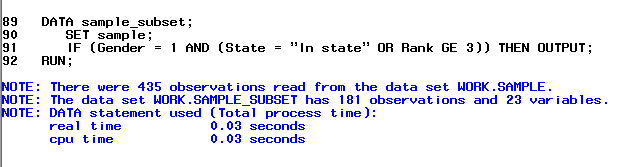### Example - Delete cases with specific conditions (numeric and character variables)

Now let’s say we want to exclude freshmen students that are also in-state students. (That means that our subset will contain all sophomores, all juniors, all seniors, all students with missing class rank values, and out-of-state freshmen.)

DATA sample_small;
SET sample;
IF (Rank = 1 AND State = "In state") THEN DELETE;
RUN;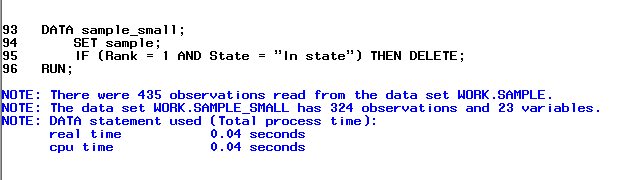### Example - Keep cases having values within a specific range

Now let's say we want to include only the observations whose Math scores fall between 55 and 75. On paper, we can write this condition using the notation $$55 \leq x \leq 75.$$ However, SAS does not recognize this notation. Instead, we must rewrite this as two conditions $$x \geq 55$$ and $$x \leq 75$$ joined with an AND statement.

DATA sample_math;
SET sample;
IF (Math GE 55 AND Math LE 75) THEN OUTPUT;
RUN;


After running the block of code, you should see that the new subset "sample_math" has 330 observations, corresponding to the number of students who met the inclusion criteria.If you want to make sure that the procedure works, you can compute the mean of the variable from the new dataset and see if the data falls between the specified range.

PROC MEANS data=sample_math;
VAR Math;
RUN;As you can see, the observations for the Math variable are within the 55 to 75 range that we specified.

## Subsetting Datasets by Rows

It's also possible to subset your data based on row position. For example, you may want to extract all cases in a dataset beginning at the 5th row, or extract the first 30 cases in a dataset, or extract rows 20 through 30 of a dataset.

The general syntax for the three types of "row extraction" is as follows:

Extract cases beginning at row i:

DATA New-Dataset-Name (OPTIONS);
SET Old-Dataset-Name (firstobs=i);
RUN;

Extract the first j rows:

DATA New-Dataset-Name (OPTIONS);
SET Old-Dataset-Name (obs=j);
RUN;

Extract rows i through j:

DATA New-Dataset-Name (OPTIONS);
SET Old-Dataset-Name (firstobs=i obs=j);
RUN;

For these examples, let's work with the following tiny set of data, so that it's easier to see which rows are extracted in each case:

DATA tiny;
INPUT Subject_ID age;
DATALINES;
1 21
2 39
3 19
4 26
5 48
6 51
7 87
8 26
9 35
10 50
;
RUN;

### Example - Extract cases from row 6 through the last row of the dataset

In this situation, we want to skip over the first rows of the dataset, and keep only the cases from row 6 onward.

DATA tiny_from_row_6;
SET tiny(firstobs=6);
RUN;

#### Log window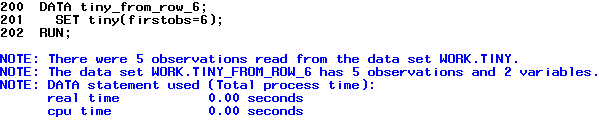#### Resulting dataset (printed using PROC PRINT)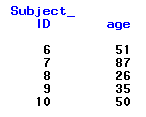### Example - Extract the first 6 rows

This situation is the opposite of the previous example: here, we want to keep the first rows of the dataset, up through and including row 6. (This implicitly means that we are extracting rows 1 through 6.)

DATA tiny_first_6;
SET tiny(obs=6);
RUN;

#### Log window#### Resulting dataset (printed using PROC PRINT)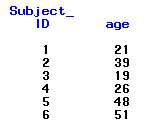### Example - Extract rows 3 through 7

This type of logic is used if you want to extract a specific sequence of rows.

DATA tiny_3_through_7;
SET tiny(firstobs=3 obs=7);
RUN;

#### Log window#### Resulting dataset (printed using PROC PRINT)In general, if you are able to express the cases you want to extract in terms of one of your variables, it's preferable to use the above IF-THEN approach, rather than telling SAS to extract by rows.

## Splitting a Dataset

Sometimes you may want to split a dataset into two or more datasets based on the value(s) of a variable(s).

In this kind of data step, you create two or more datasets at one time based on one whole dataset. This method uses conditional logic with the THEN OUTPUT keywords. It is an extension of the method described above for subsetting data. The basic code to create two datasets is as follows:

DATA New-Dataset-Name-1 (OPTIONS) New-Dataset-Name-2 (OPTIONS);
SET Old-Dataset-Name (OPTIONS);
IF (insert conditions for Dataset1) THEN OUTPUT New-Dataset-Name-1;
IF (insert conditions for Dataset2) THEN OUTPUT New-Dataset-Name-2;
RUN;

Alternatively, you may wish to partition a dataset by separating all cases with a certain criteria into one dataset, and all cases not meeting that criteria into a second dataset. This means that the cases in the two datasets will be mutually exclusive and exhaustive. The basic code to partition a dataset in this manner is:

DATA New-Dataset-Name-1 (OPTIONS) New-Dataset-Name-2 (OPTIONS);
SET Old-Dataset-Name (OPTIONS);
IF (insert conditions for Dataset1) THEN OUTPUT New-Dataset-Name-1;
ELSE OUTPUT New-Dataset-Name-2;
RUN;

Or, if using more than two criteria:

DATA New-Dataset-Name-1 (OPTIONS) New-Dataset-Name-2 (OPTIONS) New-Dataset-Name-3 (OPTIONS);
SET Old-Dataset-Name (OPTIONS);
IF (insert conditions for Dataset1) THEN OUTPUT New-Dataset-Name-1;
ELSE IF (insert conditions for Dataset2) THEN OUTPUT New-Dataset-Name-2;
ELSE OUTPUT New-Dataset-Name-3;
RUN;

You can partition a dataset in this manner into as many subsets as you want: just make sure you correctly count how many subsets will be created by your sequence of IF-ELSE statements, and be sure to specify names for each of those subsets in the DATA statement.

### Example - Splitting into multiple datasets using more than one IF statement

The general code above only shows the case where a dataset is partitioned into two datasets, but it's possible to partition a dataset into as many pieces as you wish. In the DATA statement, list the names for each of the new data sets you want to create, separated by spaces. Then in the body of the data step, you'll write an OUTPUT statement for each dataset name you've specified. Let's illustrate this by splitting the sample dataset into four parts based on class rank, creating one dataset for each class.

DATA freshmen sophomores juniors seniors;
SET sample;
IF (Rank = 1) THEN OUTPUT freshmen;
IF (Rank = 2) THEN OUTPUT sophomores;
IF (Rank = 3) THEN OUTPUT juniors;
IF (Rank = 4) THEN OUTPUT seniors;
RUN;

Note that this block of code does not take into account the cases where variable Rank is missing. That means that the splits in this example were mutually exclusive, but not exhaustive: some of the cases were lost in the split.

### Example - Splitting into two datasets using IF and ELSE statements

Now let's try to partition the dataset so that all freshmen are in one dataset, and all other cases are put in a second dataset. That means that the second dataset will contain the "not-freshmen"; i.e., sophomores, juniors, seniors, and any cases with a missing value for variable Rank. Unlike Example 1, this split will create mutually exclusive and exhaustive datasets; that is, none of the original cases will be lost in the split.

DATA freshmen not_freshmen;
SET sample;
IF (Rank = 1) THEN OUTPUT freshmen;
ELSE OUTPUT not_freshmen;
RUN;

Let's compare the first few cases of the new datasets. The new dataset called freshmen should look pretty homogenous with respect to variable Rank:By comparison, the new dataset called not_freshmen should look more diverse. Notice that observation 9 (student ID 15) has a missing value for Rank.### Example - Splitting using IF and ELSE statements and logic based on missing values

The ELSE OUTPUT statement can also be used to partition a dataset based on missing and nonmissing values. Recall that missing values are denoted by a period (.) for numeric variables, and by a blank for character variables. Let's try to partition a dataset into missing and non-missing cases with respect to the variable State, which is a character variable.

DATA is_missing not_missing;
SET sample;
IF (State = "") THEN OUTPUT is_missing;
ELSE OUTPUT not_missing;
RUN;

To indicate a missing character value, we use an empty set of quotation marks (""). (Recall that quotation marks are used in SAS to indicate strings, so having two quotation marks next to each other indicates a blank string.) The syntax IF (State="") literally says "if State is blank".

Try viewing the new dataset called is_missing. The first few rows of output should look like this: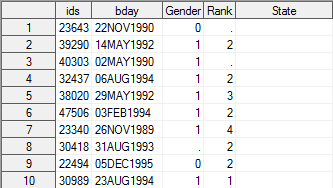You should see that the column containing the cases for State appears completely empty, indicating that all the cases in this dataset have missing observations for State.